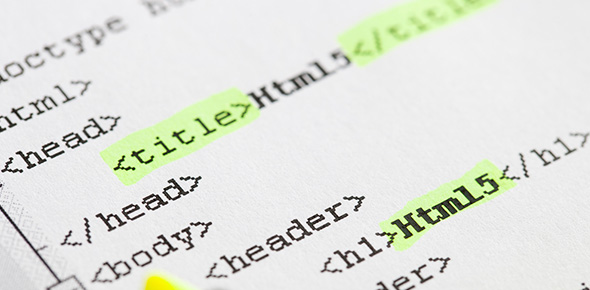# JavaScript Quiz: MCQ Trivia Exam!

20 Questions | Attempts: 371
ShareSettings• 1.
Name two different ways to call a function.
• A.

When the function is stored in the JavaScript Folder

• B.

When it is invoked from JavaScript code

• C.

When an event occurs (when a user clicks a button)

• D.

When you book a hall for a private function

• 2.
What would best describe the code below: var nums = [ 1, 2, 3, 4, 5, 6, 7, 8, 9 ];
• A.

An array of strings

• B.

An array of integers

• C.

A variable

• D.

A list of numbers

• 3.
JavaScript code will load before the webpage if stored in the head tags.
• A.

True

• B.

False

• 4.
It can be considered good practice to insert JavaScript code before the closing body ta, as this allows the HTML to load first
• A.

True

• B.

False

• 5.
When linking to an external JavaScript file what extension would the file have?
• A.

.javascript

• B.

.js

• C.

.java

• D.

.JSCRIPT

• 6.
When calling a function you pass in ......
• 7.
A user defined function or a built in function can have a list of.....
• A.

Arguments

• B.

Parameters

• C.

Functions

• D.

Variables

• 8.
In the loop below when i equals 2 what color will be displayed in the console? var colours = [red, green, blue]; for(i = 0;  i < colours.length;  i++)  { console.log(colours[i]); }
• A.

Red

• B.

Blue

• C.

Green

• D.

None of the above

• 9.
In the code below what word describes each time the loop goes round? for(i = 0;  i < 10;  i++)  { console.log( i );
• A.

Interaction

• B.

Iteration

• C.

Individual

• D.

Interstellar

• 10.
JavaScript is commonly known as...
• A.

Server Side Scripting Language

• B.

Client Side Scripting Language

• C.

Browser Scripting Language

• D.

Interactive Scripting Language

• 11.
JavaScript is used to...
• A.

• B.

Add functionality to Server Side Pages

• C.

To style webpages

• D.

To create layout and structure for webpages

• 12.
What are the variables used for?
• A.

Storing integers for manipulating in code

• B.

Storing operators for manipulating in code

• C.

Storing methods for manipulating in code

• D.

Storing Strings for manipulating in code

• 13.
When joining 2 strings together in JavaScript this is known as?
• A.

Concave

• B.

Concatenation

• C.

Convexity

• D.

Convulsion

• 14.
If a variable is created inside a function the variable scope would be known as...
• A.

Global

• B.

Cosmic

• C.

Local

• D.

Familiar

• 15.
A Global variable can be accessed from any part of your program.
• A.

True

• B.

False

• 16.
Which part of the following code is the conditional statement? if ( x > 5)  { console.log("Good Morning"); }else{"Goodnight"}
• A.

Else{"Goodnight")

• B.

( x > 5)

• C.

Document.write

• D.

If

• 17.
When Developing Software - check the boxes that would be considered good practice.
• A.

Appropriate naming of variables

• B.

Appropriate description/naming of functions

• C.

Commenting all logic in the code

• D.

Make sure you use a cool theme

• 18.
Which one of the choices below is considered a selection construct in programming?
• A.

While

• B.

If..maybe

• C.

If...else

• D.

Var array

• 19.
Which Animal will the if statement display in the alert box? var animals = [ "Dog", "Cat", "Lion", "Bird", "Duck" ]; for( i = 0; i < animals.length; i++ ) { if( i === 4) { alert("The animal name is " + animals[ i ] ); }else{console.log(animals[ i ]); } }
• A.

Cat

• B.

Lion

• C.

Bird

• D.

Duck

• E.

Dog

• 20.
Will the following condition return true OR false ?var x = 6;if( x >= 6 )  {console.log("Yes " +  x + "  is a Great Number")}
• A.

True

• B.

False

## Related TopicsBack to top
×

Wait!
Here's an interesting quiz for you.